WEBINAR
Numerical Analysis of Magnetic Pulse Welding Process
Thursday, October 5, 2023
Time
SESSION 1
SESSION 2
CEST (GMT +2)
03:00 PM
08:00 PM
EDT (GMT -4)
09:00 AM
02:00 PM
HOME / Applications / Electro-thermal simulation of parallel current carrying wires

# Electro-thermal simulation of parallel current carrying wires

Used Tools:## Parallel current carrying wires

In this example, capability of coupling analysis in EMS will be treated by a simple example of two parallel wires as shown in Figure 1. A transient magnetic simulation coupled to thermal analysis was solved. This simulation allows us to validate the transfer of EM generated ohmic heating to the thermal field.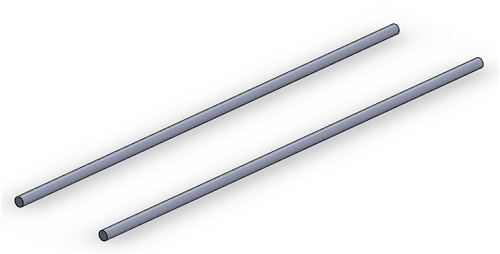Figure 1 - CAD model of simulated example

The Transient Magnetic analysis belongs to the low-frequency electromagnetic domain or regime; i.e. displacement currents are neglected. It calculates magnetic fields that vary over time. These fields are typically caused by surges in currents or voltages. This type of analysis can be linear or non-linear. It also addresses eddy currents, power losses and magnetic forces.

## Simulation setup

After creating an Transient Magnetic study coupled to thermal analysis in EMS, four important steps shall always be followed:

1. apply the proper material for all solid bodies
2. apply the necessary electromagnetic inputs
3. apply the necessary thermal inputs
4. mesh the entire model and run the solver

## Materials

In the table 1, wires material properties are listed.

Table 1 -  Material properties

 Components / Bodies Relative Permeability Electrical Conductivity Thermal Conductivity (W/m*K) Specific Heat (J/Kg*K) Masse Density (Kg/m^3) Wire Material 1 5.00e+7 390 385 8900

## Electromagnetic inputs

In this study, pulse current is applied on each wire in the same direction. Figure 2 shows the excitation current curve of the pulse.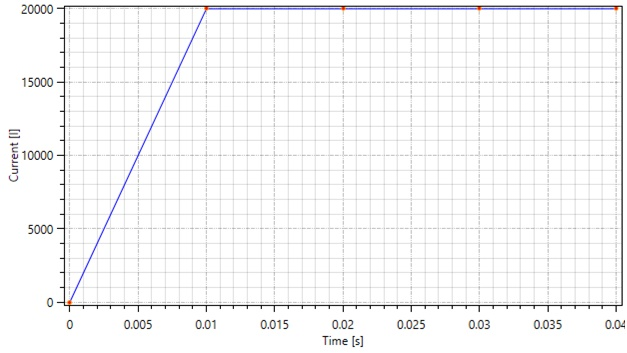Figure 2 - Current form in each wire

## Meshing

Meshing is a very crucial step in the design analysis. EMS estimates a global element size for the model taking into consideration its volume, surface area, and other geometric details. The size of the generated mesh (number of nodes and elements) depends on the geometry and dimensions of the model, element size, mesh tolerance, and mesh control. In the early stages of design analysis where approximate results may suffice, you can specify a larger element size for a faster solution. For a more accurate solution, a smaller element size may be required. Figure 3 and 4 shows the mesh details and the final mesh respectively.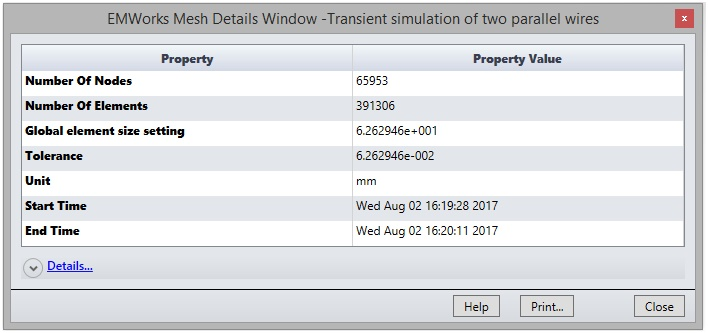Figure 3 - Mesh details of the model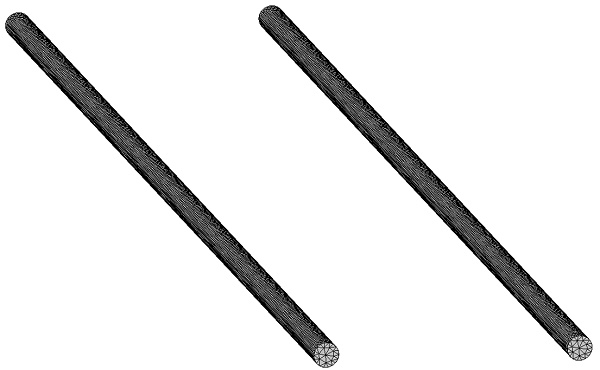Figure 4 - Meshed wires

## Electromagnetic-thermal results

After finishing the setup, a successfully run of Transient Magnetic simulation coupled to thermal analysis, generates both electric and thermal transient results. The total solving thermal time is set to 0.03 second with 0.002 second as time increment. In the figure below is a 3D plot of total current density (applied + induced current) at 0.006 second.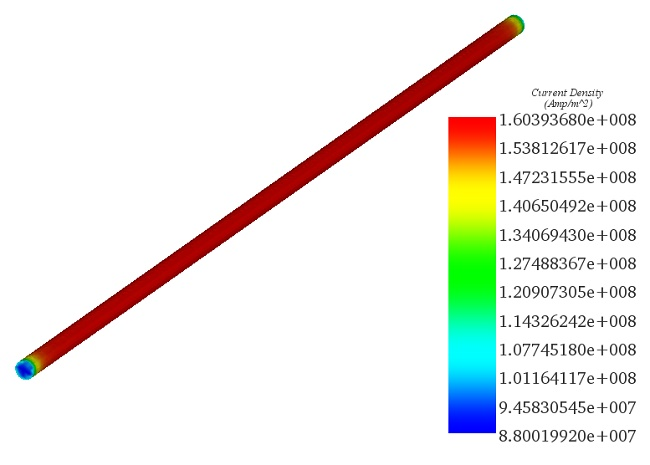Figure 5 - Distribution of total current density

The solid loss that will appear as heat in the two wires, has the same evolution behaviour of the excitation current as shown in Figure 6. So, the temperature evolution follows also the solid loss variation as shown in Figure 7. The results agree well with .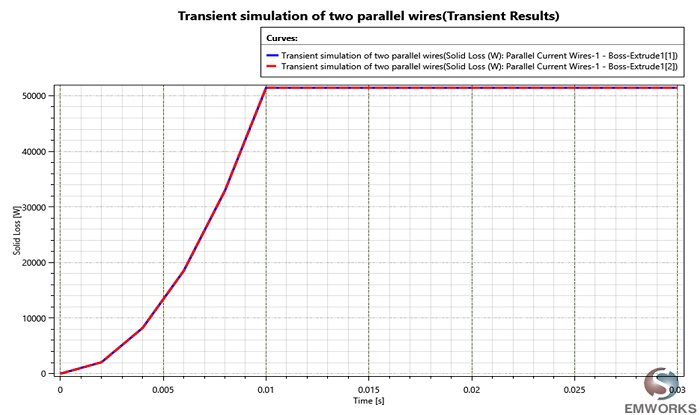Figure 6 - Evolution of Solid loss in the two wires.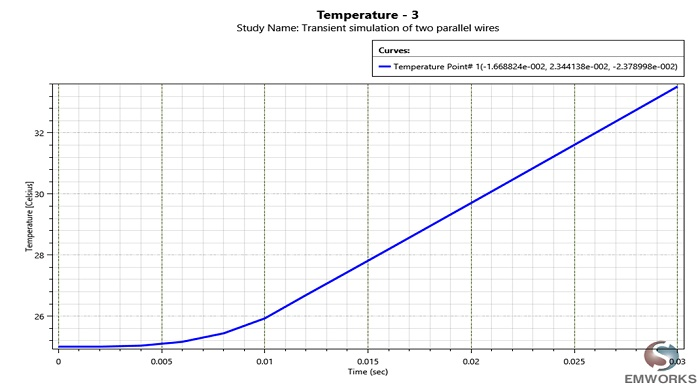Figure 7 - Temperature variation in function of time

## Conclusion

EMS can solve coupled electric – thermal problems where both the magnetic flux distribution as well as the temperature distribution can be studied. This finds many applications in high-voltage power transmission.

## References

: William Lawson and Anthony Johnson: “A simple Weak-Field Coupling Benchmark Test of the Electromagnetic-Thermal- Structural Solution Capabilities Of LS-DYNA Using Parallel Current Wires “